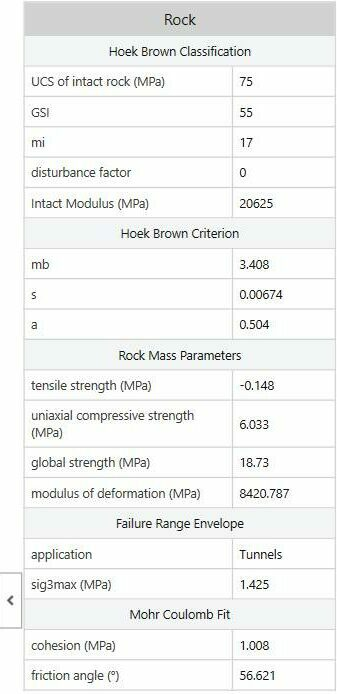The Rocscience International Conference 2021 Proceedings are now available. Read Now

# Equivalent Mohr-Coulomb Parameters

An important feature of RSData is the calculation of equivalent Mohr-Coulomb parameters for non-linear failure envelopes. This feature is available in Table values view and is very useful because many geotechnical engineering analysis programs require Mohr-Coulomb parameters (cohesion and friction angle) as input, even though actual strength envelopes are often non-linear.

The equivalent Mohr-Coulomb parameters are automatically calculated if you are working with one of the non-linear strength criteria in RSData:

Mohr-Coulomb parameters are calculated by determining a linear envelope which provides a "best-fit" over a given stress range of a non-linear envelope. The stress range is specified using the Failure range option in materials dialog.

Below shows an example of Mohr-Coulomb parameters calculated and displayed in the data table as shown below.